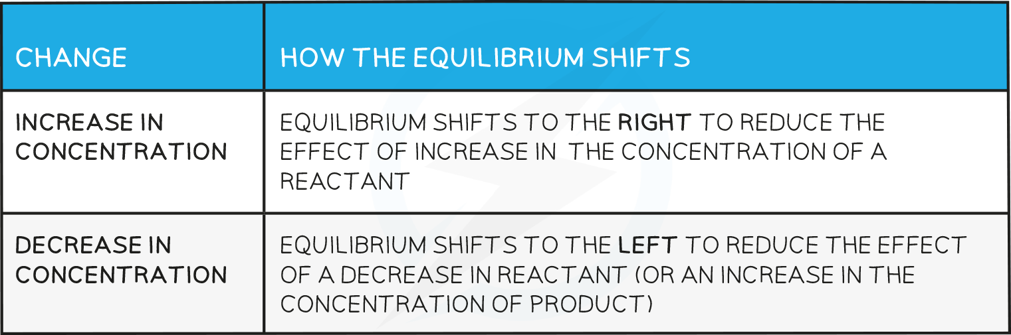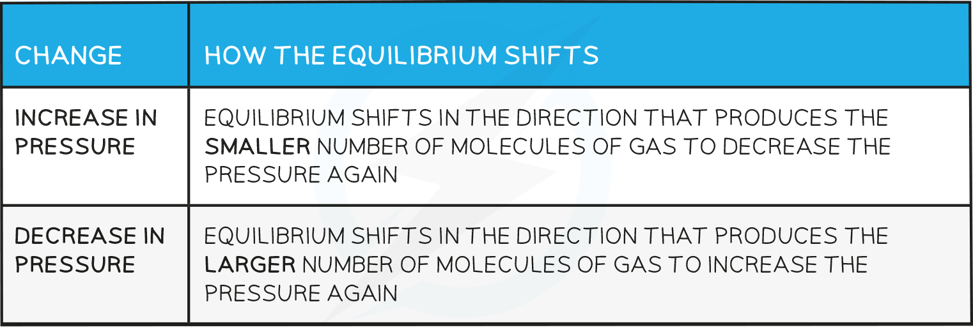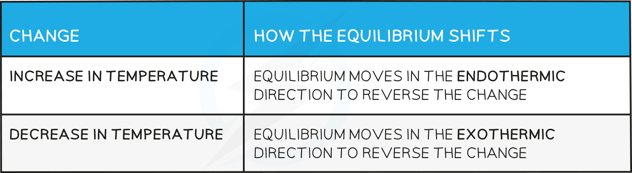# Edexcel A Level Chemistry:复习笔记1.10.2 Le Chatelier's Principle

### Le Chatelier's Principle

#### Position of the equilibrium

• The position of the equilibrium refers to the relative amounts of products and reactants in an equilibrium mixture
• When the position of equilibrium shifts to the left, it means the concentration of reactants increases
• When the position of equilibrium shifts to the right, it means the concentration of products increases

#### Le Chatelier’s principle

• Le Chatelier’s principle says that if a change is made to a system in dynamic equilibrium, the position of the equilibrium moves to counteract this change
• The principle is used to predict changes to the position of equilibrium when there are changes in temperature, pressure or concentration

#### Effects of concentration

How the equilibrium shifts with concentration changes#### Worked Example

Changes in equilibrium position

Using the reaction below:

CH3COOH (I) + C2H5OH (I)  ⇌  CH3COOC2H5 (I) + H2O (I)

Explain what happens to the position of equilibrium when:

1. More CH3COOC2H5 is added

2. Some C2H5OH is removed

Using the reaction below:

Ce4+ (aq) + Fe2+ (aq) ⇌ Ce3+ (aq) + Fe3+ (aq)

Explain what happens to the position of equilibrium when

3. Water is added to the equilibrium mixture

• The position of the equilibrium moves to the left and more ethanoic acid and ethanol are formed
• The reaction moves in this direction to oppose the effect of added ethyl ethanoate, so the ethyl ethanoate decreases in concentration

• The position of the equilibrium moves to the left and more ethanoic acid and ethanol are formed
• The reaction moves in this direction to oppose the removal of ethanol so more ethanol (and ethanoic acid) are formed from ethyl ethanoate and water

• There is no effect as the water dilutes all the ions equally so there is no change in the ratio of reactants to products

#### Effects of pressure

• Changes in pressure only affect reactions where the reactants or products are gases

How the equilibrium shifts with pressure changes#### Worked Example

Changes in pressure

Predict the effect of increasing the pressure on the following reactions:

1. N2O4 (g) ⇌ 2NO2 (g)

2. CaCO3 (s) ⇌ CaO (s) + CO2 (g)

Predict the effect of decreasing the pressure on the following reaction:

3. 2NO2 (g) ⇌ 2NO (g) + O2 (g)

• The equilibrium shifts to the left as there are fewer gas molecules on the left
• This causes a decrease in pressure

• The equilibrium shifts to the left as there are no gas molecules on the left but there is CO2 on the right
• This causes a decrease in pressure

• The equilibrium shifts to the right as there is a greater number of gas molecules on the right
• This causes an increase in pressure

#### Effects of temperature

How the equilibrium shifts with temperature changes#### Worked Example

Changes in temperature

Using the reaction below:

H2 (g) + CO2 (g) ⇌  H2O (g) + CO (g)    ΔH = +41.2 kJ mol-1

1. Predict the effect of increasing the temperature on this reaction

Using the reaction below:

Ag2CO3 (s)  ⇌  Ag2O (s) + CO2 (g)

2. Increasing the temperature increases the amount of CO2(g) at constant pressure. Is this reaction exothermic or    endothermic?

• The reaction will absorb the excess energy and since the forward reaction is endothermic, the equilibrium will shift to the right